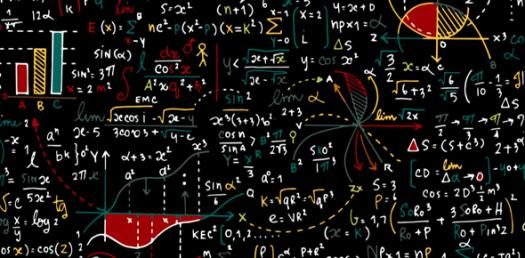# Test Your Algebra I Math Skills!

9 Questions | Attempts: 1169
ShareSettingsDo you believe that you are an algebra pro and can tackle any question on Algebra one that comes your way? If you gave a big yes to that then this test is for you. Give it a try and see if you are really the best of the best.

• 1.
Write an algebraic expression for the verbal expression: the sum of the square of a number and 34.
• A.

X+34

• B.

X^2+34^2

• C.

X^2+34

• D.

34-x^2

• 2.
Evaluate the expression when w = 4, n = 8, v = 5, and t = 2: 2nt - v^2
• A.

3

• B.

7

• C.

89

• D.

6

• 3.
(5x^3y) (12x^2y^3z)
• A.

60x^5y^4z

• B.

17x^6y^6z^2

• C.

7x^2yz^2

• D.

2x^2y^2z^2

• 4.
What are the two possible values of 0 if x^2 - 36 = 0?
• A.

{-1,7}

• B.

{-6,6}

• C.

{6,4}

• D.

{-6,1}

• 5.
Factor completely using integers: 4ax - 14bx = 35by - 10ay
• A.

(2 + 4ay) (4 - 6bx)

• B.

-10abx = 25aby

• C.

(2x - 5y) (2a - 7b)

• D.

(3x + 7y) (5a + 7b)

• 6.
Define two variables, write two equations, and solve: A movie theater sold 450 tickets, receiving a total of \$1800. If each adult ticket was \$5 and each child ticket was \$2, how many adult tickets were sold?
• A.

225

• B.

155

• C.

450

• D.

300

• 7.
Solve -z/5 - 3 < 4
• A.

Z < 7

• B.

Z = 45

• C.

Z > -35

• D.

Z < -5

• 8.
Determine the slope of the line passing through the pair of points: (6,-4) and (-3,7)
• A.

-3/4

• B.

-11/9

• C.

1/2

• D.

6

• 9.
A price increased from \$35 to \$36.40. What was the percent of increase?
• A.

4%

• B.

11%

• C.

3%

• D.

2.5%

## Related TopicsBack to top
×

Wait!
Here's an interesting quiz for you.